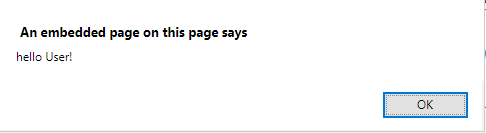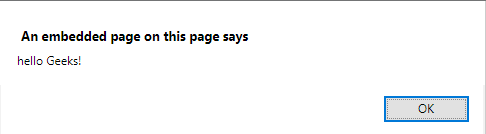Related Articles

# Passing a function as a parameter in JavaScript

• Last Updated : 02 May, 2019

Passing a function as an argument to the function is quite similar to the passing variable as an argument to the function. Below examples describes to passing a function as a parameter to another function.

Example 1: This example passes a function geeks_inner to the function geeks_outer as an argument.

 ` ``<``html``> ``    ``<``head``> ``        ``<``title``> ``            ``JavaScript | Pass a function as parameter ``        `` ``    `` ``     ` `    ``<``body` `style` `= ``"text-align:center;"``> ``         ` `        ``<``h1` `style` `= ``"color:green;"` `> ``            ``GeeksForGeeks ``        `` ``         ` `        ``<``p``> ``            ``Passing function as arguments. ``        `` ``         ` `        ````        ``<``button` `onclick` `= ``"geeks_outer(geeks_inner)"``> ``            ``Click Here ``        ``     ``         ` `        ``<``script``> ``            ``function geeks_inner(value){``                ``return 'hello User!';``            ``}``            ``function geeks_outer(func){``                ``alert(func());``            ``}``        `` ``    `` ``                    `

Output:

• Before clicking on the button:• After clicking on the button:Example 2: This example passes a function geeks_inner alongwith a argument ‘Geeks!’ to the function geeks_outer as a argument.

 ` ``<``html``> ``    ``<``head``> ``        ``<``title``> ``            ``JavaScript | Pass a function as parameter ``        `` ``    ``     ``     ` `    ``<``body` `style` `= ``"text-align:center;"``> ``         ` `        ``<``h1` `style` `= ``"color:green;"` `> ``            ``GeeksForGeeks ``        `` ``     ` `        ``<``p``> ``            ``Passing function as arguments. ``        ``             ``         ` `        ``<``button` `onclick` `= ``"geeks_outer('Geeks!', geeks_inner)"``> ``            ``Click Here ``        ``         ``         ` `        ````        ``<``script``> ``            ``function geeks_inner(value){``                ``return 'hello '+value;``            ``}``            ``function geeks_outer(a, func){``                ``alert(func(a));``        ``}         ``        `` ``    `` ``                    `

Output:

• Before clicking on the button:• After clicking on the button:Hey geek! The constant emerging technologies in the world of web development always keeps the excitement for this subject through the roof. But before you tackle the big projects, we suggest you start by learning the basics. Kickstart your web development journey by learning JS concepts with our JavaScript Course. Now at it’s lowest price ever!

My Personal Notes arrow_drop_up